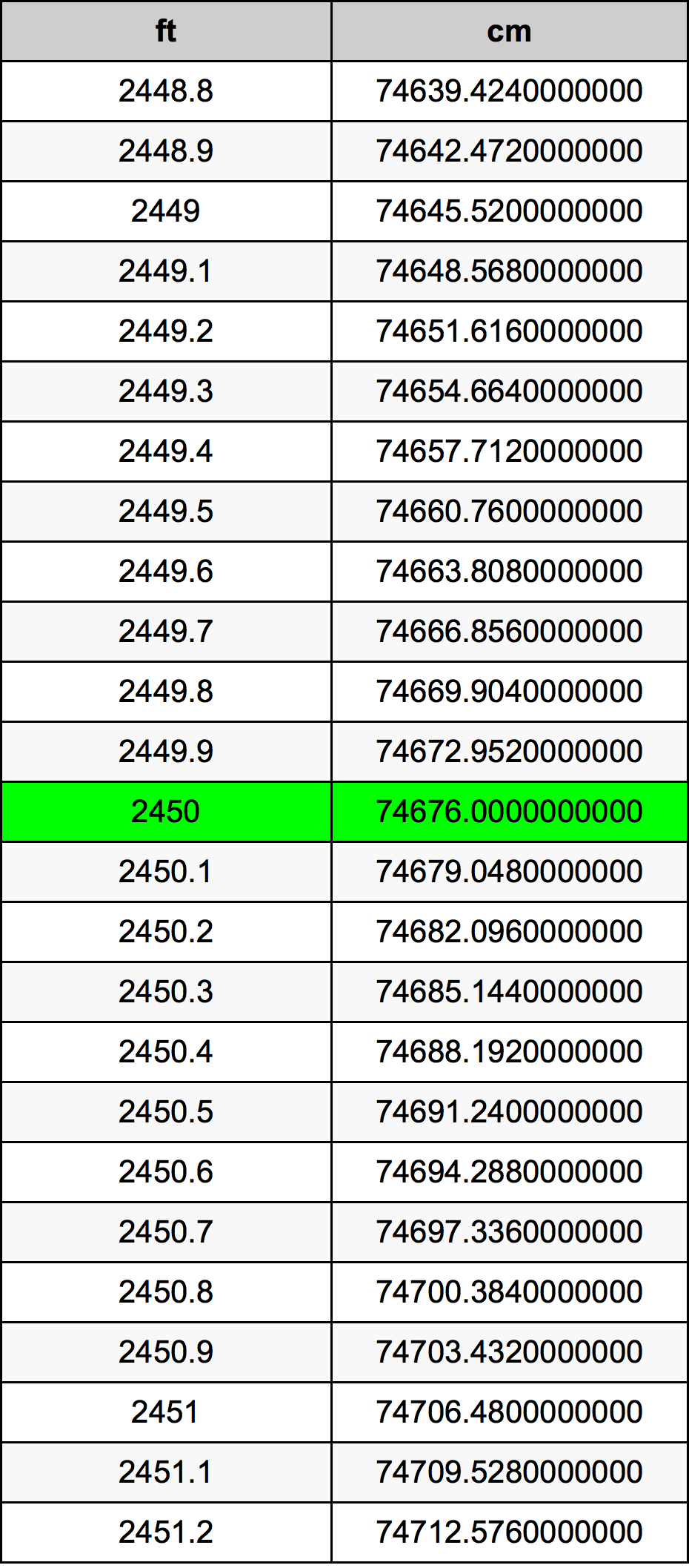Feet To Cm

# 2450 ft to cm2450 Feet to Centimeters

ft
=
cm

## How to convert 2450 feet to centimeters?

 2450 ft * 30.48 cm = 74676.0 cm 1 ft
A common question is How many foot in 2450 centimeter? And the answer is 80.3805774278 ft in 2450 cm. Likewise the question how many centimeter in 2450 foot has the answer of 74676.0 cm in 2450 ft.

## How much are 2450 feet in centimeters?

2450 feet equal 74676.0 centimeters (2450ft = 74676.0cm). Converting 2450 ft to cm is easy. Simply use our calculator above, or apply the formula to change the length 2450 ft to cm.

## Convert 2450 ft to common lengths

UnitUnit of length
Nanometer7.4676e+11 nm
Micrometer746760000.0 µm
Millimeter746760.0 mm
Centimeter74676.0 cm
Inch29400.0 in
Foot2450.0 ft
Yard816.666666667 yd
Meter746.76 m
Kilometer0.74676 km
Mile0.4640151515 mi
Nautical mile0.4032181425 nmi

## What is 2450 feet in cm?

To convert 2450 ft to cm multiply the length in feet by 30.48. The 2450 ft in cm formula is [cm] = 2450 * 30.48. Thus, for 2450 feet in centimeter we get 74676.0 cm.

## 2450 Foot Conversion Table## Alternative spelling

2450 ft to cm, 2450 ft in cm, 2450 Foot to cm, 2450 Foot in cm, 2450 ft to Centimeters, 2450 ft in Centimeters, 2450 Foot to Centimeter, 2450 Foot in Centimeter, 2450 Feet to cm, 2450 Feet in cm, 2450 ft to Centimeter, 2450 ft in Centimeter, 2450 Foot to Centimeters, 2450 Foot in Centimeters